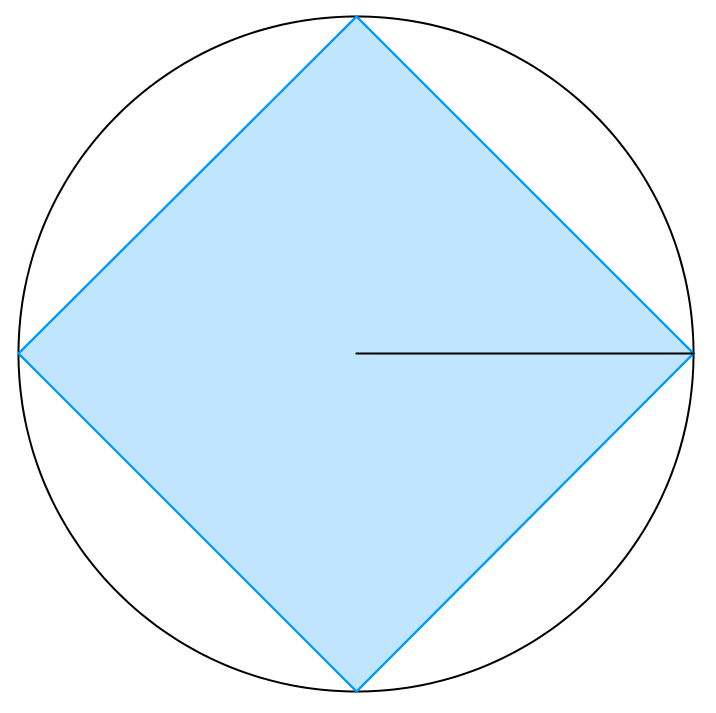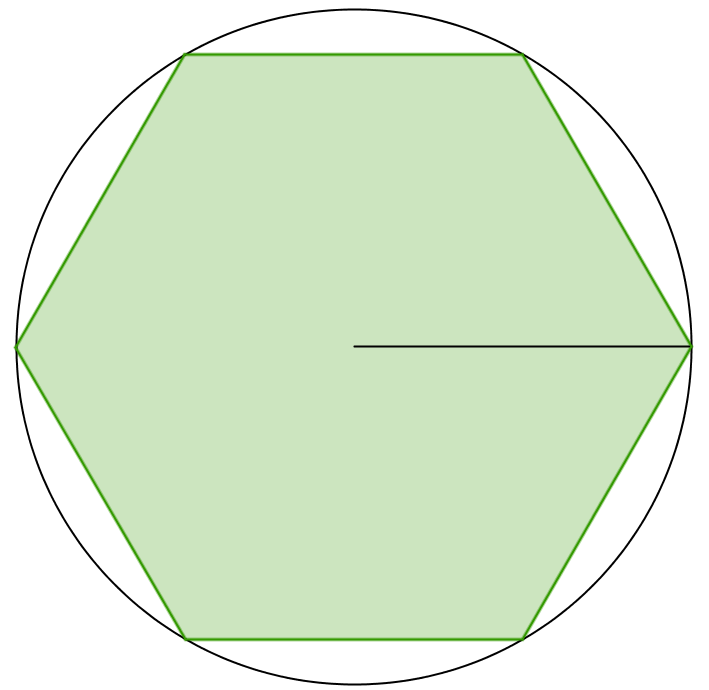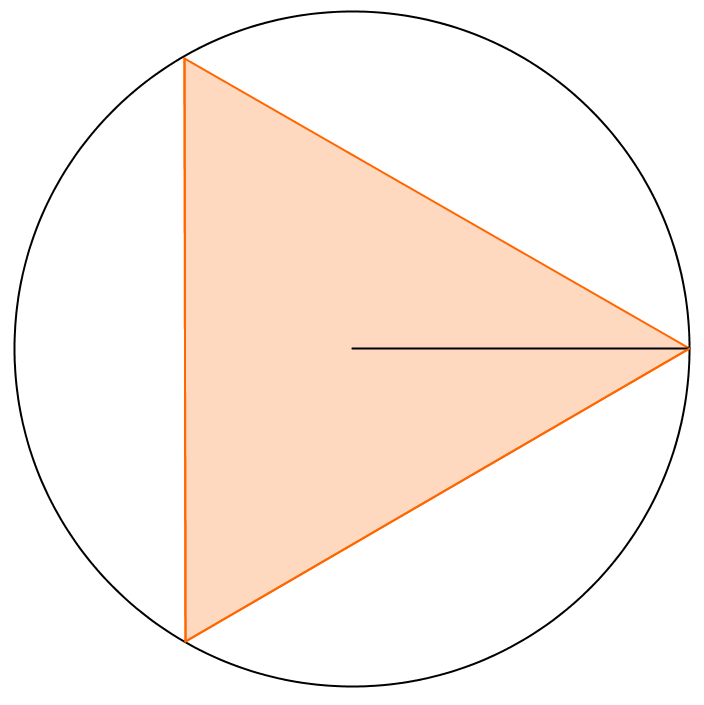You may also likeSome(?) of the Parts

A circle touches the lines OA, OB and AB where OA and OB are perpendicular. Show that the diameter of the circle is equal to the perimeter of the triangleA 1 metre cube has one face on the ground and one face against a wall. A 4 metre ladder leans against the wall and just touches the cube. How high is the top of the ladder above the ground?At a Glance

The area of a regular pentagon looks about twice as a big as the pentangle star drawn within it. Is it?

Inscribed in a Circle

Age 14 to 16Challenge Level

The area of a square inscribed in a circle with a unit radius is, satisfyingly, $2$.What is the area of a regular hexagon inscribed in a circle with a unit radius?What is the area of an equilateral triangle inscribed in a circle with a unit radius?